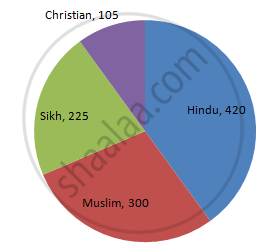Share

# Employees of a Company Have Been Categorized According to Their Religions as Given Below: - Mathematics

Course
ConceptCircle Graph Or Pie Chart - Drawing Pie Charts

#### Question

Employees of a company have been categorized according to their religions as given below:

 Religions Hindu Muslim Sikh Christian Total Number of workers 420 300 225 105 1080

Draw a pie-chart to represent the above information.

#### Solution

We know:
Central angle of a component = (component value / sum of component values × 360ο)
Here, total number of workers = 1050
Thus, the central angle for each component can be calculated as follows:

 Religion Number of workers Sector angle Hindu 420 420/1050 ×× 360 = 144 Muslim 300 300/1050 ×× 360 = 102.9 Sikh 225 225/1050 ×× 360 = 77.14 Christian 105 105/1050 ×× 360 = 36

Note: The total number of workers is 1050, not 1080.

Now, the pie chat that represents the given data can be constructed by following the steps below:
Step 1 : Draw circle of an appropriate radius.
Step 2 : Draw a vertical radius of the circle drawn in step 1.
Step 3 : Choose the largest central angle. Here, the largest central angle is 144o. Draw a sector with the central angle 144o in such a way that one of its radii coincides with the radius drawn in step 2 and another radius is in its counter clockwise direction.
Step 4 : Construct other sectors representing other items in the clockwise direction in the descending order of magnitudes of their central angles.
​Step 5 : Shade the sectors with different colours and label them as shown as in the figure below.​​Is there an error in this question or solution?

#### APPEARS IN

RD Sharma Solution for Mathematics for Class 8 by R D Sharma (2019-2020 Session) (2017 to Current)
Chapter 25: Data Handling-III (Pictorial Representation of Data as Pie Charts or Circle Graphs)
Ex. 25.1 | Q: 2 | Page no. 12

#### Video TutorialsVIEW ALL 

Solution Employees of a Company Have Been Categorized According to Their Religions as Given Below: Concept: Circle Graph Or Pie Chart - Drawing Pie Charts.
S